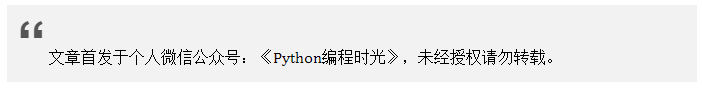# 1.24 Python 炫技操作：条件语句的七种写法¶## 原代码¶

if age > 18:
return "已成年"
else:
return "未成年"


## 第一种¶

<on_true> if <condition> else <on_false>


>>> age1 = 20
>>> age2 = 17
>>>
>>>
>>> msg1 = "已成年" if age1 > 18 else "未成年"
>>> print msg1

>>>
>>> msg2 = "已成年" if age2 > 18 else "未成年"
>>> print msg2

>>>


## 第二种¶

<condition> and <on_true> or <on_false>


>>> msg1 = age1 > 18 and "已成年" or "未成年"
>>> msg2 = "已成年" if age2 > 18 else "未成年"
>>>
>>> print(msg1)

>>>
>>> print(msg2)



## 第三种¶

(<on_true>, <on_false>)[condition]


>>> msg1 = ("未成年", "已成年")[age1 > 18]
>>> print(msg1)

>>>
>>>
>>> msg2 = ("未成年", "已成年")[age2 > 18]
>>> print(msg2)



## 第四种¶

(lambda: <on_false>, lambda:<on_true>)[<condition>]()


>>> msg1 = (lambda:"未成年", lambda:"已成年")[age1 > 18]()
>>> print(msg1)

>>>
>>> msg2 = (lambda:"未成年", lambda:"已成年")[age2 > 18]()
>>> print(msg2)



## 第五种¶

{True: <on_true>, False: <on_false>}[<condition>]


>>> msg1 = {True: "已成年", False: "未成年"}[age1 > 18]
>>> print(msg1)

>>>
>>> msg2 = {True: "已成年", False: "未成年"}[age2 > 18]
>>> print(msg2)



## 第六种¶

((<condition>) and (<on_true>,) or (<on_false>,))


>>> msg1 = ((age1 > 18) and ("已成年",) or ("未成年",))
>>> print(msg1)

>>>
>>> msg2 = ((age2 > 18) and ("已成年",) or ("未成年",))
>>> print(msg2)